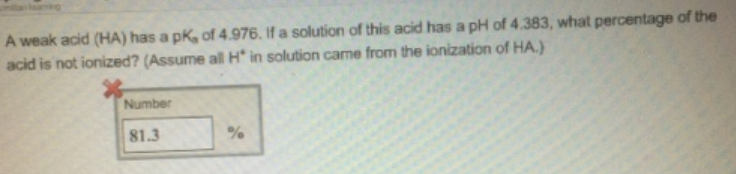# Problem: A weak acid (HA) has a pKa of 4.976. If a solution of this acid has a pH of 4.383, what percentage of the acid is not ionized? (Assume all H+ in solution came from the ionization of HA).

###### FREE Expert Solution
87% (385 ratings)###### Problem Details

A weak acid (HA) has a pKa of 4.976. If a solution of this acid has a pH of 4.383, what percentage of the acid is not ionized? (Assume all H+ in solution came from the ionization of HA).